[BACK]
 Computer Systems Science & EngineeringDOI:10.32604/csse.2023.023120Article

Improved Load-Balanced Clustering for Energy-Aware Routing (ILBC-EAR) in WSNs

1Department of Electronics and Communication Engineering, Info Institute of Engineering, Coimbatore, 641107, India
2Department of Computer Science and Engineering, SNS College of Technology, Coimbatore, 641035, India
*Corresponding Author: D. Loganathan. Email: loguudt@gmail.com
Received: 28 August 2021; Accepted: 07 December 2021

Abstract: Sensors are considered as important elements of electronic devices. In many applications and service, Wireless Sensor Networks (WSNs) are involved in significant data sharing that are delivered to the sink node in energy efficient manner using multi-hop communications. But, the major challenge in WSN is the nodes are having limited battery resources, it is important to monitor the consumption rate of energy is very much needed. However, reducing energy consumption can increase the network lifetime in effective manner. For that, clustering methods are widely used for optimizing the rate of energy consumption among the sensor nodes. In that concern, this paper involves in deriving a novel model called Improved Load-Balanced Clustering for Energy-Aware Routing (ILBC-EAR), which mainly concentrates on optimal energy utilization with load-balanced process among cluster heads and member nodes. For providing equal rate of energy consumption among nodes, the dimensions of framed clusters are measured. Moreover, the model develops a Finest Routing Scheme based on Load-Balanced Clustering to transmit the sensed information to the sink or base station. The evaluation results depict that the derived energy aware model attains higher rate of life time than other works and also achieves balanced energy rate among head node. Additionally, the model also provides higher throughput and minimal delay in delivering data packets.

Keywords: Wireless sensor networks; energy consumption; load balanced clustering; finest routing

1  Introduction

In recent scenario of technological development, Wireless Sensor Networks are widely adopted in several applications such as medical services, military surveillance, object detection, industries and object tracking and so on. The sensors are observing the data from the deployed area, process them and forward to the sink. The sensor node contains units such as sensing unit, processing unit, storage unit, power supply and communication links. Moreover, the communications are processed with single or multi-hop packet transmissions . The major confrontation in WSN is the resource limitations, such as power and storage. Hence, there is a requirement for increasing the network lifetime . For prolonging the network longevity, energy efficiency and load balancing are the significant metrics that are to be focussed. Those metrics provide, balanced and minimized energy consumption between sensor nodes. In methods based on achieving energy efficiency, the nodes in the energy efficient routes are used frequently and those nodes may exhaust earlier. Additionally, because of many-to-one communications, the sensors nearer to the sink node may loss more energy than others. This is because of the unbalanced pattern of energy consumption . The Fig. 1 depicts the general process of sensor network communication to transmit the sensed information through the optimal network.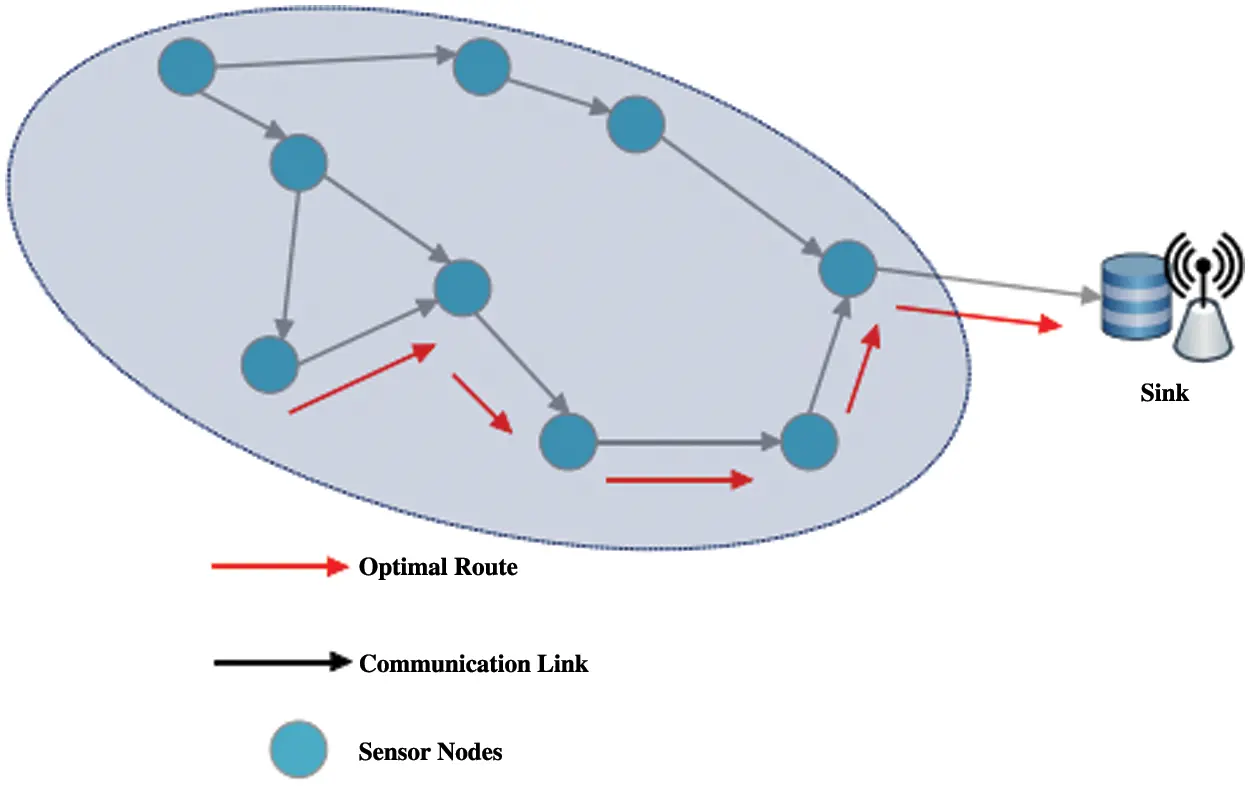Figure 1: Data transmission through optimal route

Here, the rate of energy consumption between sensors is to effectively manage to prolong the network longevity. Additionally, the sensor network communications require reliable data transmission to the sink node in many to one pattern [6,7]. Hence, energy efficiency in the network model is considered as the major challenge in WSN. On the other hand, unbalanced energy consumption makes several issues on framing efficient routing. In WSN, the data transmission and reception are assumed as the major factor in measuring the rate of energy consumption [8,9]. In several routing models, the construction of optimal route and energy consumption process influences on the WSN lifetime. For solving the issues, clustering techniques are effectively used. And, the contributions of the proposed model is listed below,

•   Defining an uneven clustering pattern for reducing and balancing the energy consumption rate of all Head_Sensors of each cluster

•   The cluster dimensions are measured to calculate the distance between the Head_Sensors for effective route determination.

•   Deriving a Finest Routing Scheme (FRS) using minimal hop communications and reduced delay

•   Based on the minimal hop-level based packet transmission, uneven clustering pattern is framed for balancing energy consumption rate, thereby enhancing network longevity.

•   For providing scalable and fault tolerant model, the sensor node densities are balanced in all clusters.

•   Model evaluation based on metrics such as Energy Efficiency, Network longevity, throughput, packet delivery rate and transmission delay.

The remainder of this paper is framed as follows; Section 2 provides the works or related studies. The network model and the work procedure of proposed techniques are presented in Section 3. In Section 4, the obtained results are expounded and comparison charts are provided for evidencing the model efficiency. At last, the paper is completed with the Conclusion and Future work in Section 5.

2  Related Work

This section presents the description of several model involved in providing efficient sensor network communications. The well-known model is LEACH , in which the cluster heads are selected in accordance with the probability rates of their thresholds for being heads. However, the limitations in LEACH are given as,

•   The node’s residual energy was not considered for processing

•   It has been considered that all sensors can link with the sink node

For increasing the network longevity, DECSA model has been proposed in . The work used multi-hop data transmission for communication between nodes and clusters. Furthermore, the cluster heads are selected based on the residual energy of nodes and distance to base station. The drawbacks of the model are considered as the model frames small clusters in random manner. And, easy energy loss has occurred because of rapid processing of data aggregation and more amount of signal reception at the cluster node. In , the authors proposed Battery Aware Reliable Clustering (BARC) for providing load balanced model that balances the cluster size. The model helps in enhancing network lifetime, but, it imposed additional network load for propagating additional data packets.

The work presented in  derived a model for receiver conflict based mixed transmission model for energy management. Additionally, the distance and the remaining node energy of the receivers are measured based on the scalability of links and the number of neighbour sensors. The major drawback observed in the model is increasing delay in transmission of packets. A valuable work has been provided in , analysing various clustering models that divides the network for avoiding the overlapping of clusters. Moreover, for clustering, reliability, suitability and security are considered. Further, a Type-2 Fuzzy Logic (T2FL)  technique has been developed for effective clustering with increased network reliability and reduced consumption rate. A multi-hop routing model has been proposed in  for increasing lifetime of network. For constructing the route, the metrics such as remaining energy of nodes, sensor locations and hops of network region.

Power efficient routing model based on node clusters has been presented in . The authors of  derived a reliable model for energy efficient data communication in large scale wireless sensor networks. In that, doughnut shaped clusters are framed with the nodes that are in same distance from the base station. Artificial Bee Colony optimization algorithm is used for cluster head selection in that work. In , Harmony Search Algorithm based Clustering Protocol (HSACP) has been proposed for reducing the intra-cluster distances between the cluster members for optimizing the energy allocation. The model in  presented a Particle Swarm Optimization (PSO) based energy efficient routing for sensor networks. The efficiency of multi-level model has been derived in , based on the factors such as node amount and network levels. The hierarchical based data aggregation has been processed to provide optimality in WSN.

Energy Efficient Adaptive Overlapping Clustering (EEAOC) model  has been derived for continuous node observation in WSN. Moreover, dynamic cluster model has been developed in the model for data aggregation without cluster overlaps. Compressibility-based Clustering Algorithm (CBCA)  has been presented a hierarchical data aggregation process. The network topology is changed into logical chain and spatial connection between the nodes is utilized for compressive clustering. For model validation, the simulations are carried out. Moreover, in , heuristic based model has been derived for framing clusters with inactive sensor selection. The stochastic sleep scheduling model is given for node selection process, considering the range of network coverage. For providing energy efficiency and distribution of CH nodes, heuristic crossover operators are used [24,25].

The survey has provided the following findings:

•   The cluster design can be hierarchical.

•   There could be delay as a key factor in network design .

•   The spatial and temporal communication can be learnt over time.

3  Proposed System

In this work, Improved Load-Balanced Clustering for Energy-Aware Routing (ILBC-EAR) is proposed for improving the communication efficiency and network lifetime in WSN. Here, the sensing region is considered to be in circular plane. The sensor nodes that are distributed in the circular sensing region can be noted as the Head_Sensors and Member_sensors of any cluster. Since, it is assumed that the area is circular, the base station (BS) is at the center of the region as presented in Fig. 2. The nodes are deployed in random manner. The complete operations involved in the proposed model and the work flow is presented in Fig. 3. Moreover, the model comprises of the following phases,

1.    Energy Model Derivations

2.    Cluster Construction

4.    Data Collection Process

5.    Finest Route Scheme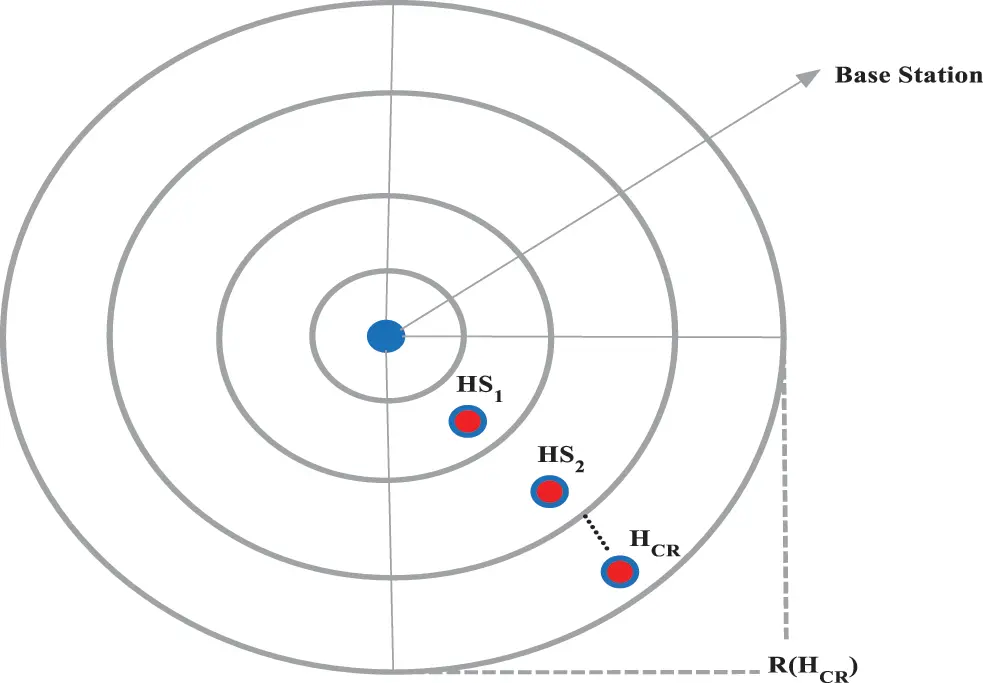Figure 2: Clustering model with concentric circle pattern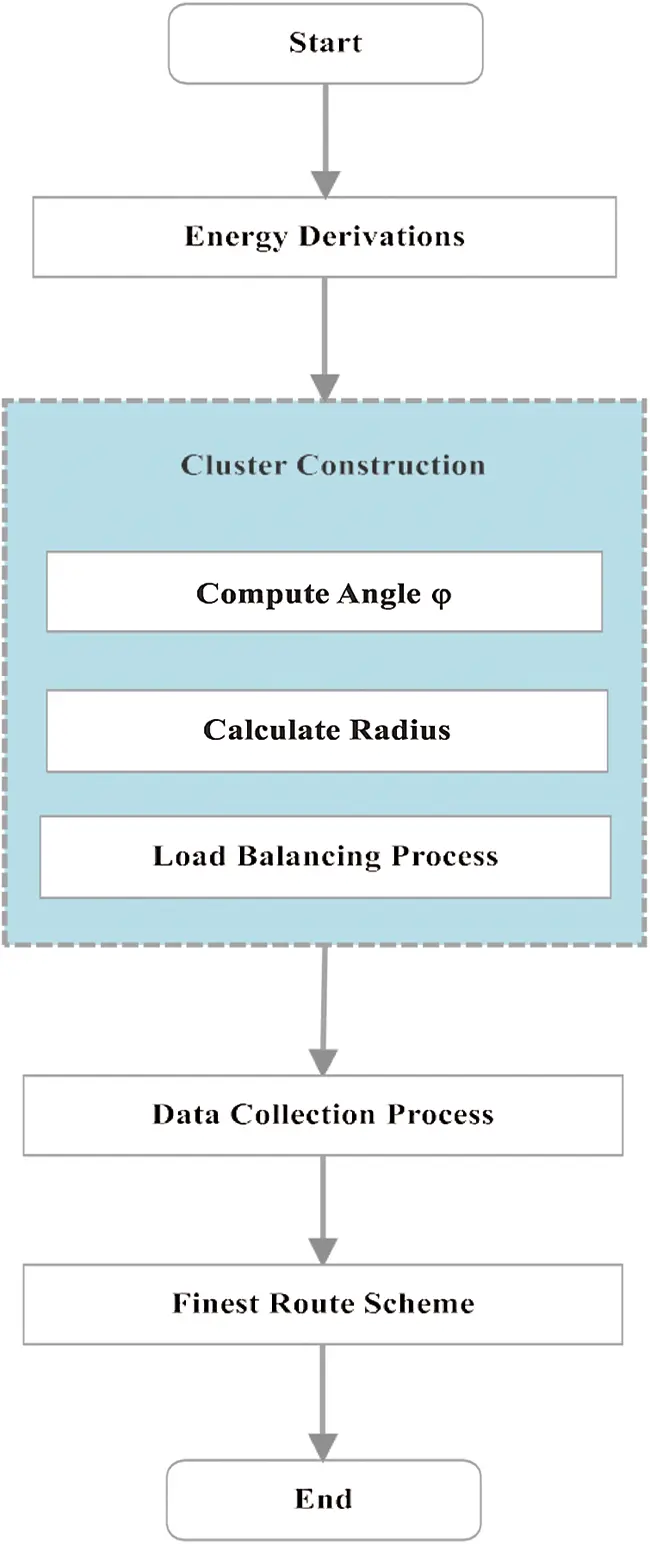Figure 3: Work flow of proposed

3.1 Energy Model Derivation

Here, the radio model is considered for deriving the rate of Energy Consumption (REC) by the deployed sensors. With respect to the distance between the transmitting and the receiving sensor, the free space and multi-path channels are employed. The free space (fs) method is used if the sensor distance is less than the threshold (sd0), or else, the multi-path technique will be utilized. Hence, the energy utilized for transmitting ‘m’ bits of data (l) for distance ‘sd’ is computed as,

ETX(l,sd)=ETXec(l)+ETXamp(l,sd)={lEec+lδfssd2ifsd<sd0lEec+lδmpsd4ifsdsd0 (1)

where ‘Eec’ the energy depletion rate occurred in electronic circuit, ‘δfs’ and ‘δmp’ represents the rate of energy consumption at ‘fs’ and energy used by multi-path channels. Further, the energy utilized for receiving ‘m’ bits are computed as in (2).

(m)=Eecm (2)

3.2 Cluster Construction

As in Fig. 2, the network area is splitd into number of clusters based on circular pattern. The complete process and the computations based on the circular radius and angle between the two concentric circles are as below.

In the above Fig. 2, the HCR is the highest rate of cluster and ‘R’ is the radius of the concentric circle. The responsibility of each head sensor of clusters is to transmit the data packets after obtaining that from member sensors (MS). The head sensors of clusters that are nearer to the base station are considered to be the route-path, since it is being the convergence to the BS. Additionally, for transmitting the observed data, the route-path framed by the head sensors are used, thereby, the data are sent to the base station. But, it is also to be considered that there are more energy is consumed by the HSs that are farer from the base station. Hence, it is significant to balance the load among the head sensors in each cluster. The circle connect distance increases more in relationship to relativity of node movement. This can be largely seen with nodes that are optimal. The convergence condition for H indicates that the values are minimal. Though this is correct it can be minimal as the node distance becomes large.

The clustering process is carried out for a single time in the network in static and permanent manner. The central computations are determined with the base station that includes the overall network design and the base stations are considered. Additionally, the base station is assumed as rechargeable unit; hence, the clustering process is carried out with that. The first step is to compute the angle φ, which is measured between the diameters of the concentric circle. For that, the longest distance between the sensors (sn) of a HS and a cluster is given as, ‘ (max(sdsnHS)) ’, which can be equal to the value of CR of each cluster. In similar manner, for all clusters, each sn is capable of sending data to the HS through the one-hop distance based data transmission. Moreover, the maximal rate of (sdHS) is occurred in the cluster at the highest rating of cluster, HCR.

Based on the Eq. (8), the angle φ is determined, with respect to the radius of concentric circle in area of network region. For obtaining the HCR value to calculate the number of clusters in the network region, the sequential numbers are measured as {i = 1, 2, 3,…}, where HCR = i in the Eq. (7). The first value through which the derivation results Ri is similar or higher than the radius (RHCR), which can be the result of HCR. Using the Eq. (6) given below, the values of φ and HCR, for all the circles are computed and hence, the clustering process is effectively accomplished.

For balancing the energy consumption of head sensors in each cluster and for maintaining the network lifetime balanced, the hop length of data transmission towards the BS from each head is calculated. The amount of data packets that are broadcasted over the network can affect the rate of energy consumption (REC) by the HSs. Based on that, the hop_length (HL) for each HS is computed as in (3).

HLiHS=CRHSHCRi+1 (3)

Additionally, the HL of head sensor of cluster with rating ‘i’ (HSi) is equal to the distance between HSi and the head sensor of the cluster with rate i-1 on the route-path to the base station, since, the data transmitted from HSi are received and transmitted by the head sensor. The clustering mechanism aggregates based on the route path. The route parameters are based on the Hop Length. The Hop length starts from 1 and continues to reach its maximum limit. As it reaches one third of the cluster distance the HL increases in unit steps of distance and converges. The length between the cluster_center ‘i’ and the base station sdHSiBS is derived from the following Eq. (4), in which Ri is the radius of ‘i’ th concentric circle, ‘φ’ is the angle between the diameters as in Fig. 2. Hence, the equation is given as,

sdHSiBS={2RiSinφ23.φ2,i=1Ri1+Ri2,i1 (4)

Then, the relation between the circle radius and communication rage (CR) is calculated as

sdHSiHSi1=RiRi22 (5)

sdHSiHSi1=CRHSHCRi+1 (6)

Using the above equations, the radius Ri and in, HCR = i, the radius value of the highest rating of cluster is determined.

Ri={CRHS(A+m=1(i12)2HCR2m),foriodd2CRHS(A2+1HCR+m=1(i2)1HCR(2m1)),forieven (7)

where, A=3φ4sinφ2HCR

By fixing the head sensors in the cluster centers, in each cluster, the longest distance between the MS and HS of cluster, ‘(max (sdHS))’, is estimated to the 1/2 of the length of cluster curve. Random Number of Nodes will not affect the finest route scheme. This is because the parameter A can be dependent on the radius that is suboptimal. Here cluster area spitted in equal space but number of nodes can vary and will not affect the performance. Assume the cluster area to be increased more than threshold the model will not degrade with performance. The highest possible rate for this distance is happened at the largest cluster circle, which is computed as,

max(sdsnHS)=φ2.RHCR (8)

In this approach the interference will be minimal because the cluster distance is fixed based on the hop length. The parameters H is optimal and can be varied based on the number of clusters.

3.4 Data Collection Process

After performing the efficient cluster construction, the data collected process is carried out for sensing the environment. The Head Sensors (HS) receives the data from the member sensors (MS). In the process of data collection, both inter and intra cluster communication is processed, in which, the data are sensed by MS and transmitted to their corresponding HS, further, the collected data in the head sensors are transmitted to the base station effectively in allotted time frame. Moreover, the sensor nodes are put in sleep mode in the operations performed by other nodes in their respective time series. The data aggregation process is carried out in HS from all the collected information, which is finally transmitted to the base station through the finest route given in next section. Unlike the member sensors in each cluster, the heads are to be in active mode all the time, causes more energy consumption by the HS. For balancing that, the cluster head responsibility is provided to other nodes in rotational manner provides energy aware processing. The total energy consumed by the HS, when the process of data collection from MS is given by,

Etot=Ems:hs+Ehs:hs (9)

where, ‘Ems:hs’ denotes the energy consumed for intra cluster communication, that is, between the MS and HS, and, ‘Ehs:hs’ denotes the energy consumption rate in inter cluster communications, that is between one HS and another. Further, ‘Ems:hs’ is derived as,

Ems:hs=ERX+EDA+EMS (10)

where, ‘ERX’ is the energy consumed at the time of data reception, ‘EDA’ is the energy utilized for data aggregation process and ‘EMS’ denotes the energy spent by member sensors under a particular HS.

EMS=i=1nj=1kETX(snj,HSi) (11)

In the above equation, ‘k’ denotes the number of nodes with a specific cluster, ‘n’ is the total number of clusters and ‘ETX(snj, HSi)’ is the energy used for data transmission from sensor ‘snj’ to its HS in ‘i’ th cluster.

EDA=m.k.Edb (12)

where, ‘m’ is the number of bits, ‘Edb’ is the energy used for aggregation of one data bit.

3.5 Finest Route Scheme

Each sensor node involves in performing sensing operation and transmitting to their corresponding head sensors. As mentioned earlier, the clusters are created in concentric circle pattern, in which the transmission between the MS and HS are considered to be in single-hop manner. Then, as given in Section 3.4, data aggregation is carried out to transmit to the base station. The following Fig. 4 demonstrates the process of finest routing making the sensed data to reach the BS. Moreover, in the process of transmitting data, each HS receives the data from the HS with its higher rating in the route path and forwards the data to the HS with lower rating in the route path. Hence, each HS obtains from a single source_node and forwards the data to a single target. Additionally, for avoiding interference, the data transmission in different route path that are carried out in different phases, thus, no interference occurred. Hence, the model is effectively performs the energy aware communication between the nodes in WSN. And, the pseudo code of the proposed model is provided in the following Tab. 1.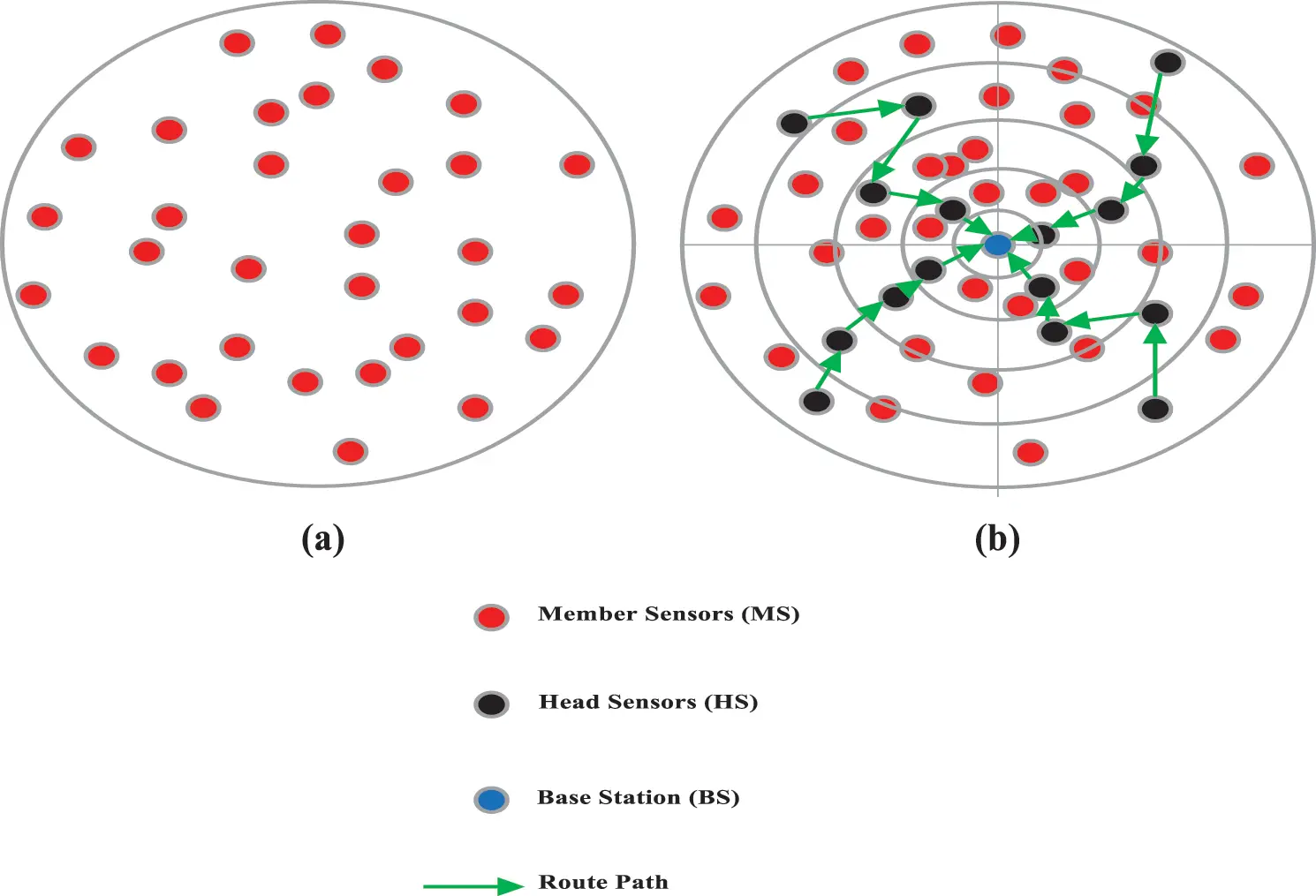Figure 4: Improved Load-Balanced Clustering for Energy-Aware Routing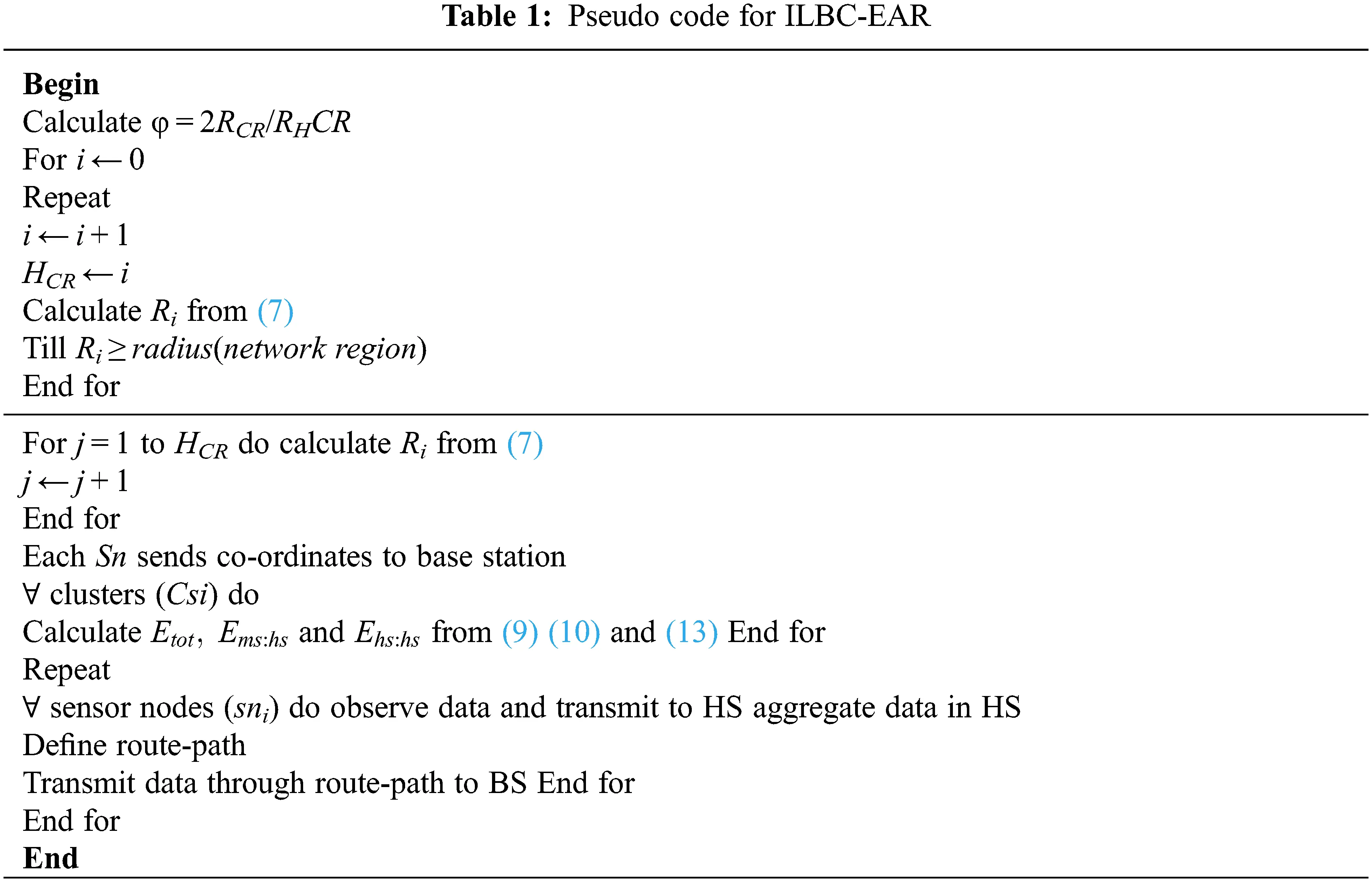4  Performance Evaluation and Discussions

For evaluating the performance of the proposed model, implementation is processed using NS-2 simulator with the simulation parameter settings provided in Tab. 2. Here, it is considered that there are 500 sensor nodes are deployed in terrain area 200 × 200 m2. For proving the efficiency of the proposed Improved Load-Balanced Clustering for Energy-Aware Routing (ILBC-EAR) model, the computed results are compared with the existing works such as, Energy Efficient Adaptive Overlapping Clustering (EEAOC) and Energy Aware Efficient Data Aggregation (EAEDAR) and Data Re-Scheduling model. Furthermore, the evaluations are carried out based on the performance analysis metrics such as Throughput, Packet Delivery Rate (PDR), End-to-End Delay, Network Lifetime and Energy Efficiency, in terms of number of nodes and payload size are shown in Figs. 5 and 6.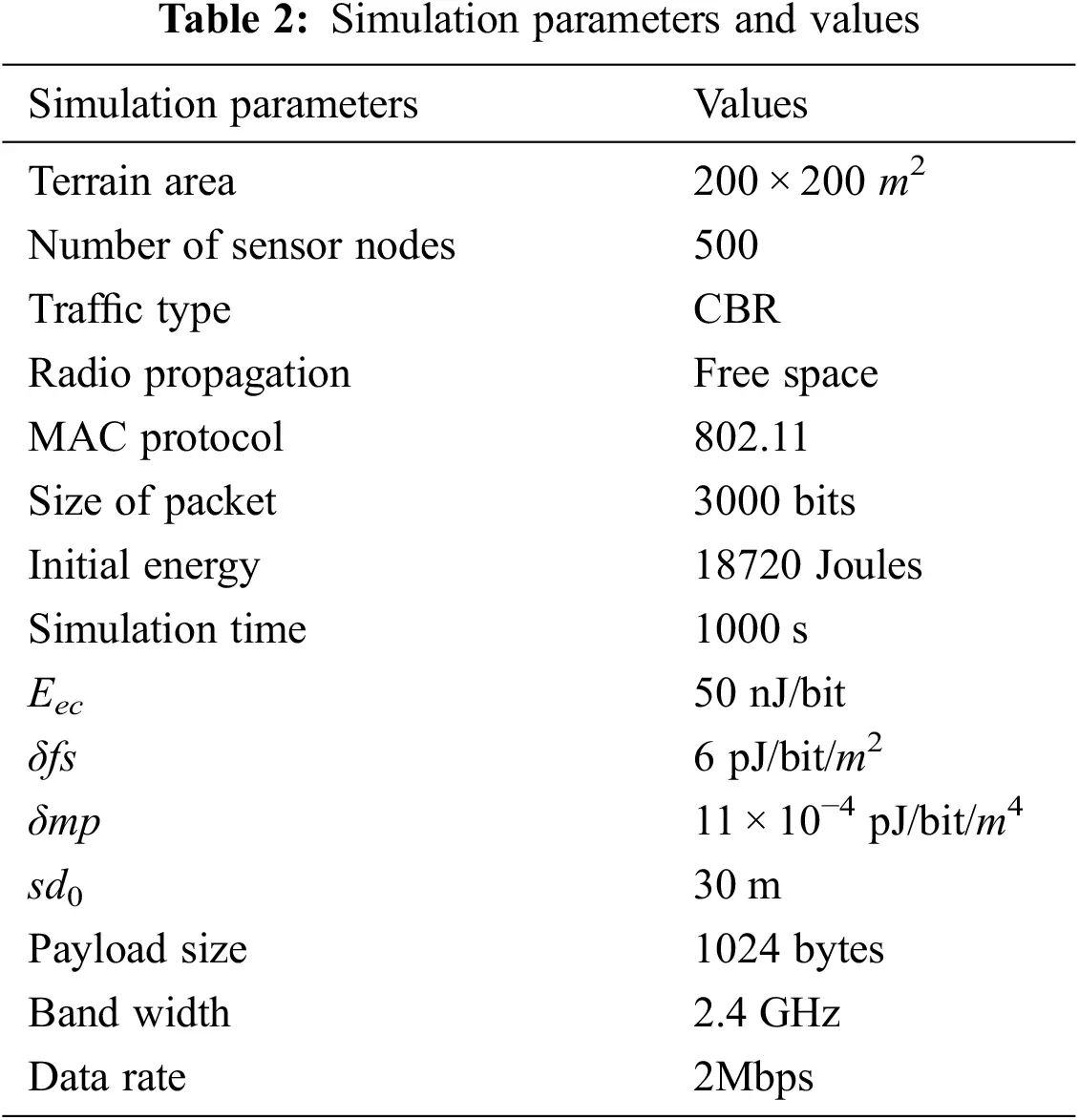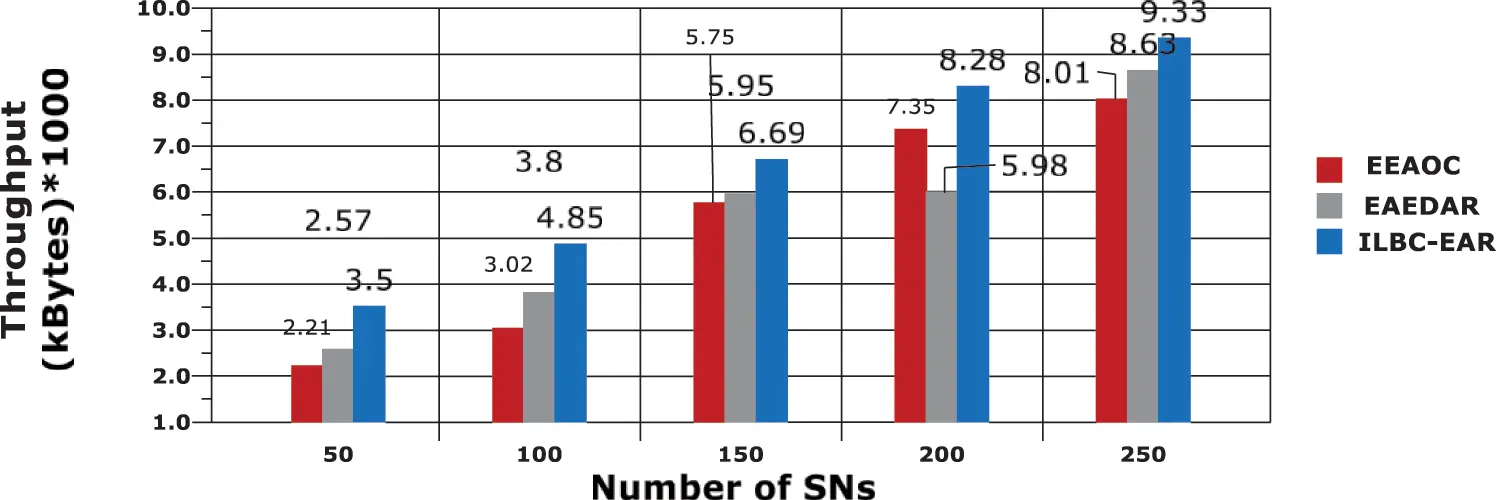Figure 5: Throughput vs. SNs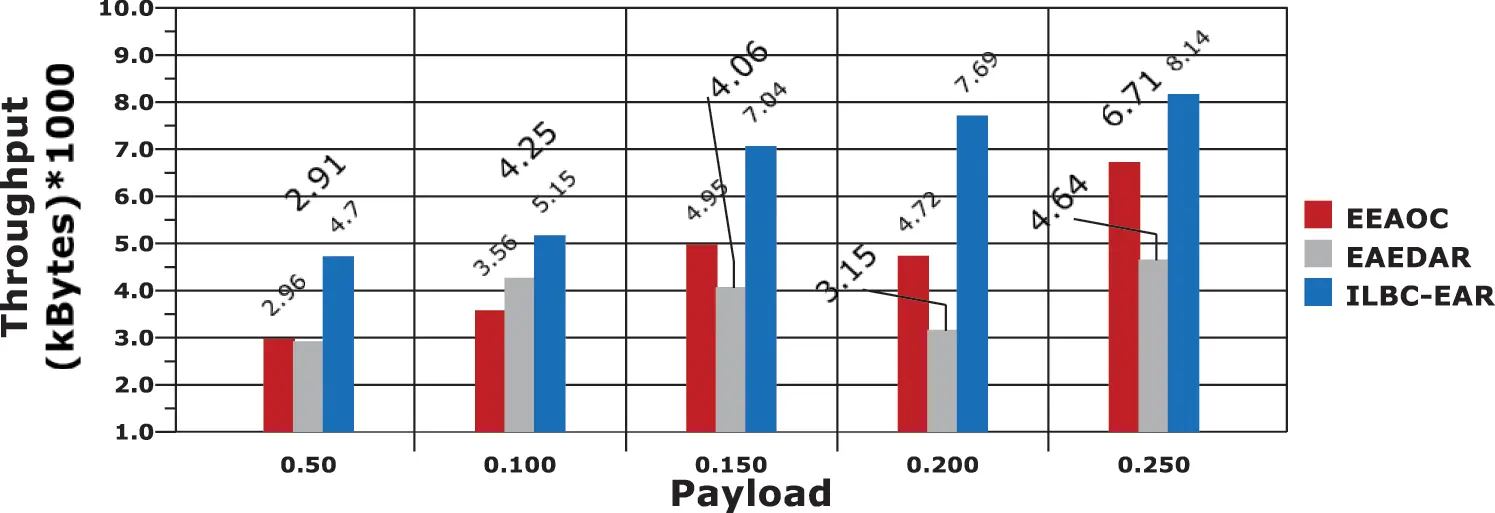Figure 6: Throughput analysis based on varying payload

In the proposed work, concentric circle based cluster formation is processed for effective route determination and achieves minimal rate of energy consumption. The model obtains throughput rates 6.53 and 6.544 in average, while analyzing with node and payload evaluations, respectively.

End-to-End Delay is evaluated here to measure the processing delay in data transmission in forwarding the sensed data to the BS. In an efficient communication model in WSN, the delay must be minimal. Here, the comparative evaluation results are presented in Figs. 7 and 8, for varying number of nodes and payload values. It is obvious from the comparisons that the ILBC-EAR model attains minimal delay than other compared works. The throughput has been improved with varying packet loss.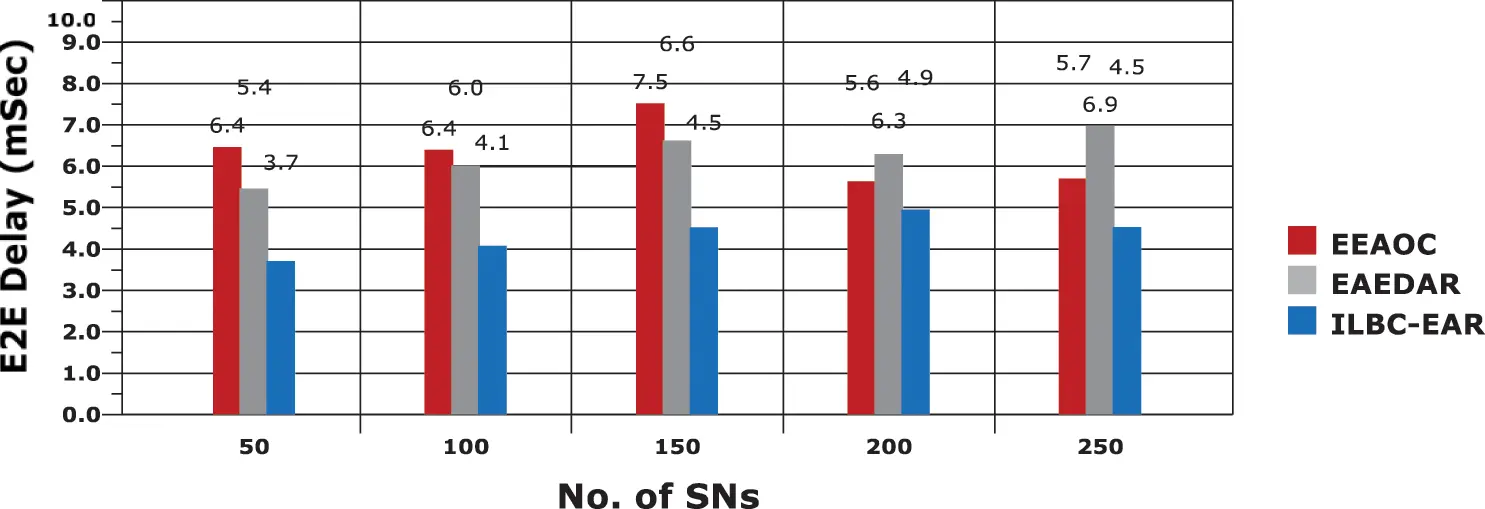Figure 7: Delay based analysis with respect to SNs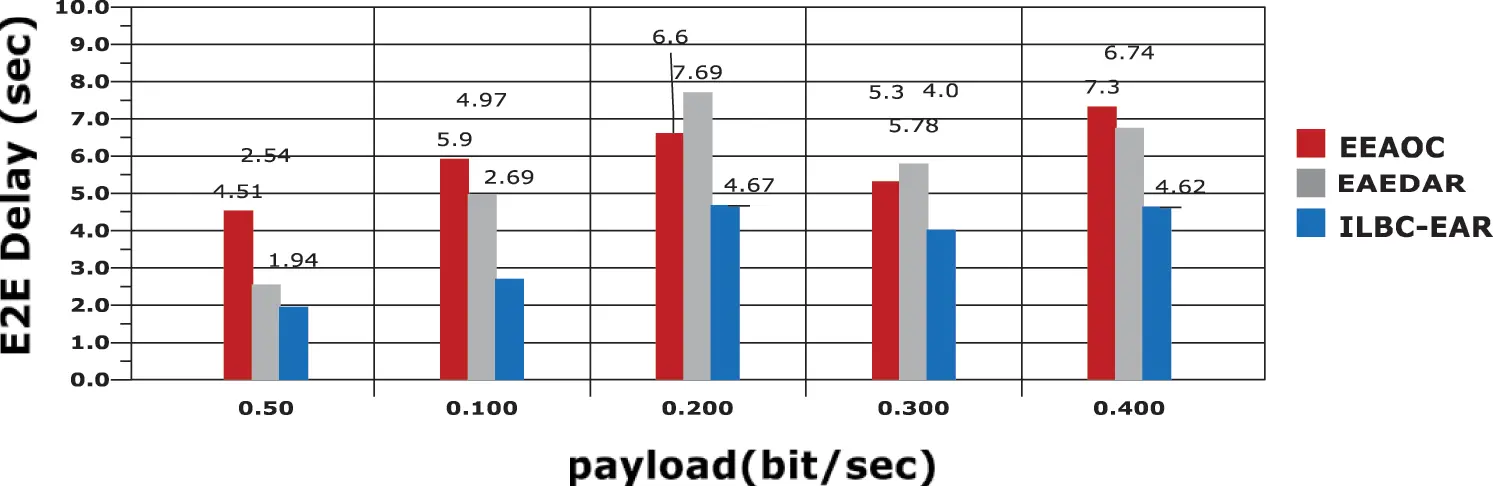Figure 8: Delay based analysis with respect to payload values

Another significant performance analysis metric is Network lifetime and the results are provided in Figs. 9 and 10, for sensor node and payload based computations, respectively. In the proposed work, the model is designed in such a manner to effectively utilize the node energy in effective manner.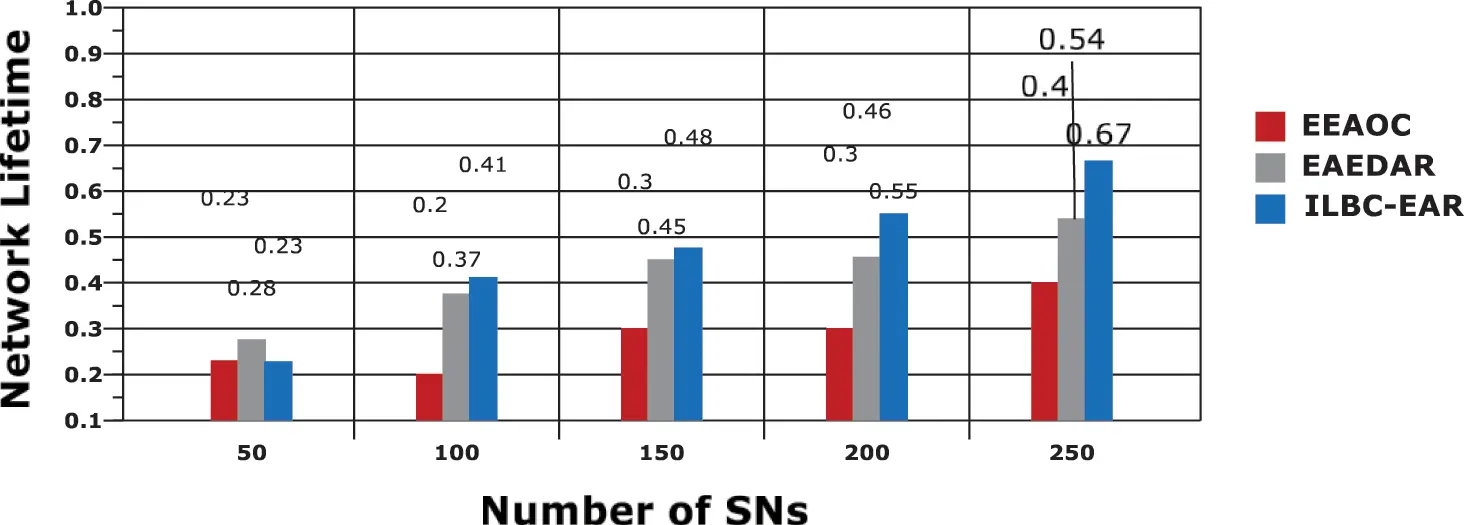Figure 9: Network lifetime vs. sensor nodes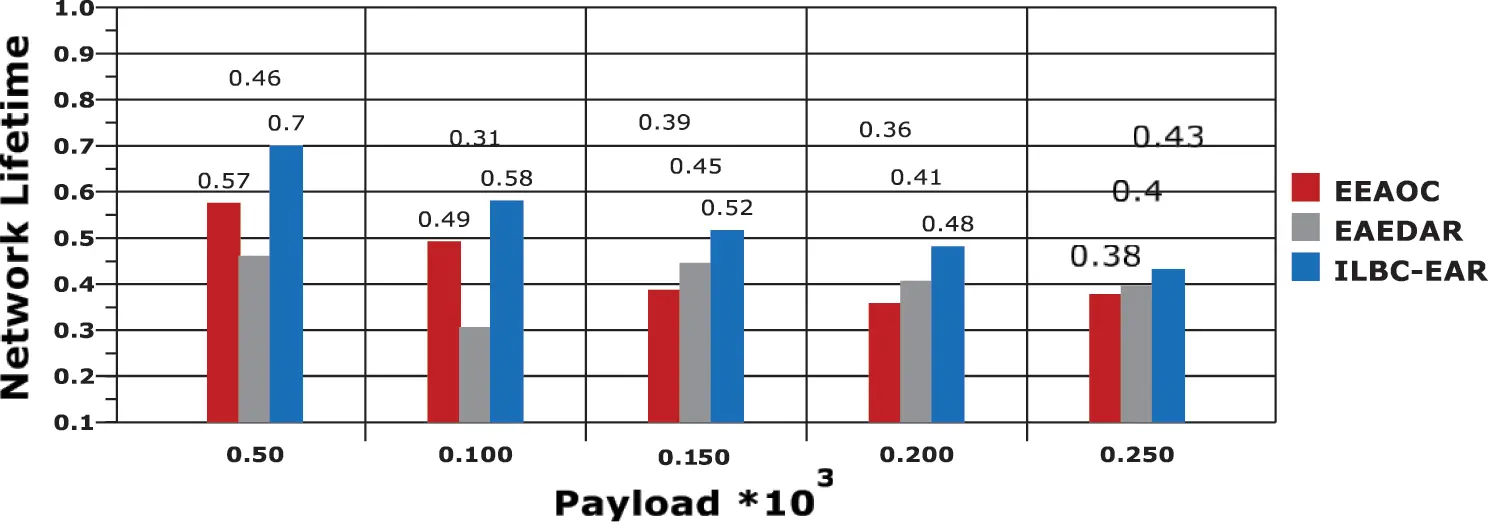Moreover, the nodes are considered to be in sleep mode, when it is not involved in a time slot of data transmission. It is observed from the following figures that the proposed work achieves higher network lifetime in both the cases. It is also explicit that the proposed model the network lifetime is inversely proportional to the increase in payload size.

The main factor in this work is energy efficiency, which is evaluated on the basis of Rate of Energy Consumption (REC) based on the number of nodes and payload size. The corresponding results are presented in Figs. 11 and 12, and the REC is measured with Joules. By balancing the load among nodes, the unwanted energy losses are effectively avoided, thereby, in ILBC-EAR, the rate of energy consumption among nodes and the overall network is effectively reduced. In the Fig. 11, in considering 250 sensor nodes in process, the average REC attained is 3.692 J, which is minimal than other compared models. Hence, the model is evidenced as energy efficient in transmitting the sensed data to the base station.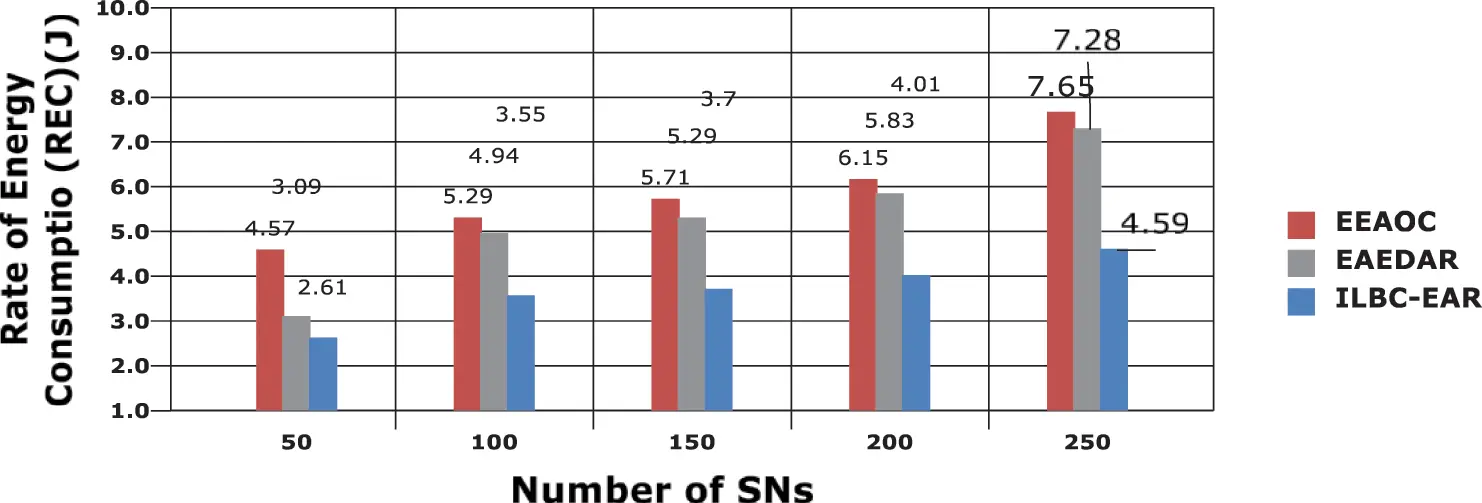Figure 11: REC evaluations with respect to SNs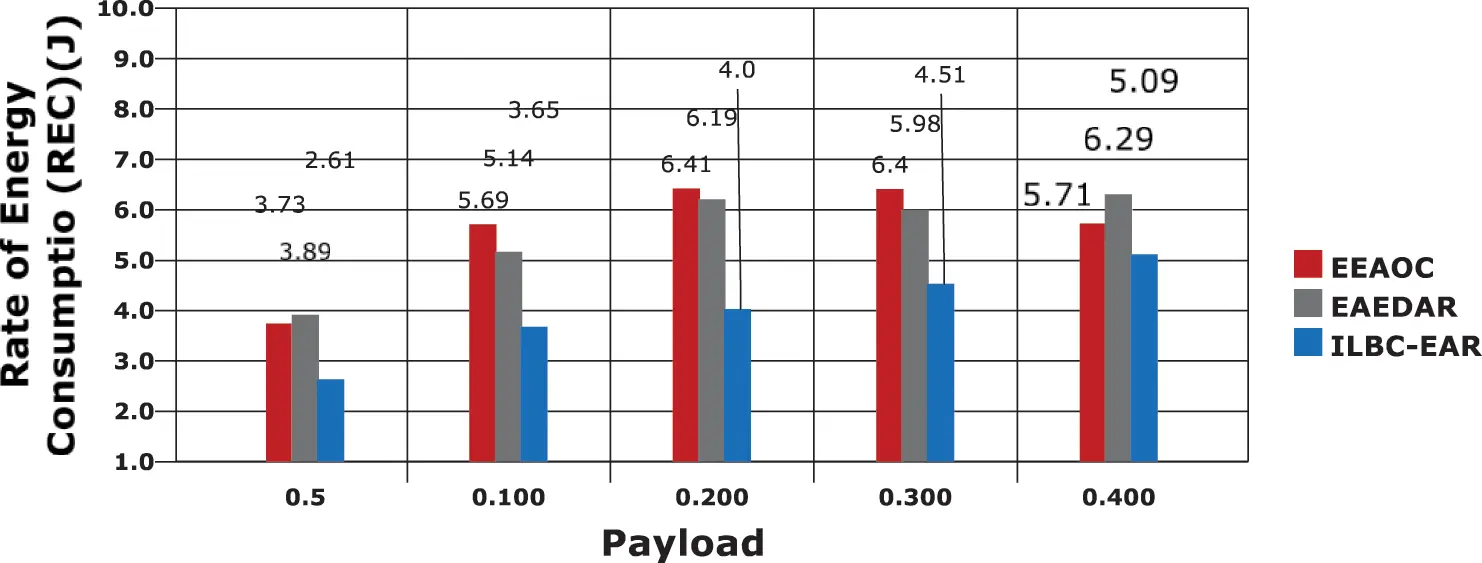Figure 12: REC Computations based on payload rate

Packet Delivery Rate (PDR) is computed here to analyse the successful rate of data packet delivery in the proposed model. And, the evaluation is processed with respect to time, and the graph is presented in Fig. 13. As it is explicit in the Fig. 13, the PDR value obtained with the affirmed work is greater than the other compared works. Moreover, it can be understood from the graph that when time increases, the PDR rate decreases, because of the increase in energy depletion of nodes. However, the proposed model provides better results than the compared works.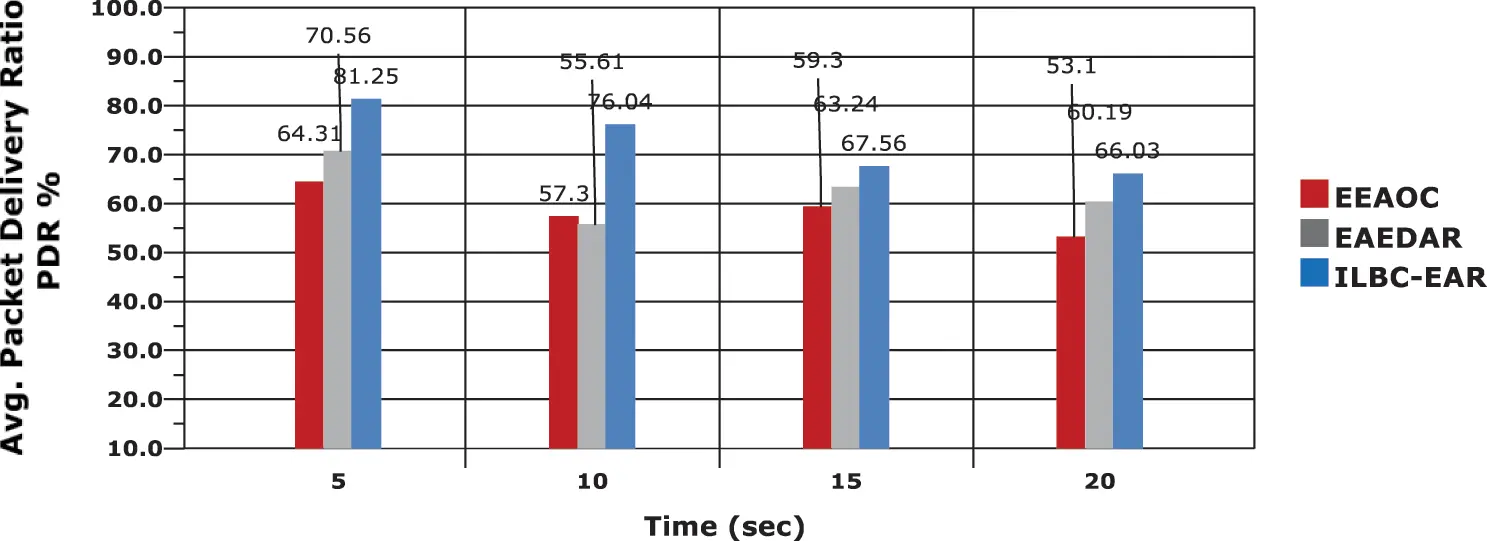Figure 13: PDR base analysis and results

5  Conclusion

This paper presents an Improved Load-Balanced Clustering for Energy-Aware Routing (ILBC-EAR) model for reducing the energy consumption rate and increasing network longevity. In this model, the sensing region is considered to be circular dimensions and the clusters are constructed as concentric circles concerning the amount of data sensed by the SNs and the data that are transmitted to the BS through the head sensors. Moreover, load balancing among nodes in the clusters is processed for avoiding unwanted energy depletion. And, finest route is framed through the head sensors for effectively transmitting the sensed data to the base station with minimal REC. The result evaluations are carried out based on energy efficiency, network lifetime, throughput, delay and PDR. The results show that the proposed model outperforms the compared works effectively.

In future, the model can be enhanced and modified to implement effectively in real-time dynamic environment. And, in other direction, more reliability can be provided with security measures in future.

Funding Statement: The authors received no specific funding for this study.

Conflicts of Interest: The authors declare that they have no conflicts of interest to report regarding the present study.

## References

1. H. A. M. Fahmy, “Wireless sensor networks: Concepts, applications, experimentation and analysis,” Signals and Communication Technology, 1st edn Berlin: Springer, 2016.
2. R. M. Curry and J. C. Smith, “A survey of optimization algorithms for wireless sensor network lifetime maximization,” Computers Industrial Engineering, vol. 101, pp. 145–166, 2016.
3. A. S. Rostami, M. Badkoobe, F. Mohanna, H. Keshavarz, A. A. R. Hosseinabadi et al., “Survey on clustering in heterogeneous and homogeneous wireless sensor networks,” Journal Super Computer, vol. 74, no. 1, pp. 277–323, 2018.
4. J. Roselin, P. Lath and S. Benitta, “Maximizing the wireless sensor networks lifetime through energy efficient connected coverage,” Ad Hoc Network, vol. 62, no. C, pp. 1–10, 2017.
5. J. Kulshrestha and M. Mishra, “An adaptive energy balanced and energy efficient approach for data gathering in wireless sensor networks,” Ad Hoc Network, vol. 54, no. C, pp. 130–146, 2017.
6. A. Ghaffari and A. Rahmani, “Fault tolerant model for data dissemination in wireless sensor networks,” in 2008 Int. Symposium on Information Technology, Kuala Lumpur, Malaysia, pp. 1–8, 2008.
7. A. Ghaffari, A. Rahmani and A. Khademzadeh, “Energy-efficient and QoS-aware geographic routing protocol for wireless sensor networks,” IEICE Electronics Express, vol. 8, pp. 582–588, 2011.
8. W. R. Heinzelman, A. Chandrakasan and H. Balakrishnan, “Energy-efficient communication protocol for wireless micro sensor networks,” in 2000 Proc. of the 33rd Annual Hawaii Int. Conf. on System Sciences, Maui, HI, USA, vol. 2, 2000.
9. S. Jamali, L. Rezaei and S. J. Gudakahriz, “An energy-efficient routing protocol for MANETs: A particle swarm optimization approach,” Journal of Applied Research and Technology, vol. 11, pp. 803–812, 2013.
10. Z. Yong and Q. Pei, “An energy-efficient clustering routing algorithm based on distance and residual energy for wireless sensor networks,” Procedia Engineering, vol. 29, pp. 1882–1888, 2012.
11. M. K. Watfa, O. Mirza and J. Kawtharani, “BARC: A battery aware reliable clustering algorithm for sensor networks,” Journal of Network and Computer Applications, vol. 32, no. 6, pp. 1183–1193, 2009.
12. J. Kulshrestha and M. Mishra, “An adaptive energy balanced and energy efficient approach for data gathering in wireless sensor networks,” Ad Hoc Network, vol. 54, no. C, pp. 130–146, 2017.
13. A. Cenedese, M. Luvisotto and G. Michieletto, “Distributed clustering strategies in industrial wireless sensor networks,” IEEE Transactions Industry Information, vol. 13, no. 1, pp. 228–237, 2017.
14. P. Nayak and B. Vathasavai, “Energy efficient clustering algorithm for multi-hop wireless sensor network using type-2 fuzzy logic,” IEEE Sensors Journal, vol. 17, no. 14, pp. 4492–4499, 2017.
15. J. Huang, Y. Hong, Z. Zhao and Y. Yuan, “An energy-efficient multi-hop routing protocol based on grid clustering for wireless sensor networks,” Cluster Computing, vol. 20, no. 4, pp. 3071–3083, 2017.
16. A. A. A. Ari, B. O. Yenke, N. Labraoui, I. Damakoa and A. Gueroui, “A power efficient cluster-based routing algorithm for wireless sensor networks: Honeybees swarm intelligence based approach,” Journal Network Computer Application, vol. 69, pp. 77–97, 20
17. S. H. Moon, S. Park and S. J. Han, “Energy efficient data collection in sink-centric wireless sensor networks,” Computer Communication, vol. 101, no. C, pp. 12–25, 20
18. D. C. Hoang, P. Yadav, R. Kumar and S. K. Panda, “Real-time implementation of a harmony search algorithm-based clustering protocol for energy-efficient wireless sensor networks,” IEEE Transactions Industry Information, vol. 10, no. 1, pp. 774–783, 2014.
19. P. Kuila and P. K. Jana, “Energy efficient clustering and routing algorithms for wireless sensor networks: Particle swarm optimization approach,” Engineering Applied Artificial Intelligence, vol. 33, pp. 127–140, 2014.
20. D. Phanish and E. J. Coyle, “Application-based optimization of multi-level clustering in Ad Hoc and sensor networks,” IEEE Transactions Wireless Communication, vol. 16, no. 7, pp. 4460–4475, 2017.
21. Y. Hu, Y. Niu, J. Lam and Z. Shu, “An energy-efficient adaptive overlapping clustering method for dynamic continuous monitoring in WSNs,” IEEE Sensors Journal, vol. 17, no. 3, pp. 834–847, 2017.
22. K. C. Lan and M. Z. Wei, “A Compressibility-based clustering algorithm for hierarchical compressive data gathering,” IEEE Sensors Journal, vol. 17, no. 8, pp. 2550–2562, 2017.
23. M. O. Oladimeji, M. Turkey and S. Dudley, “HACH: Heuristic algorithm for clustering hierarchy protocol in wireless sensor networks,” Applied Soft Computing, vol. 55, pp. 452–461, 2017.
24. K. M. Baalamurugan, S. Priyamvada Singh and R. Vijay, “A novel approach for brain tumor detection by self-organizing map (SOM) using adaptive network based fuzzy inference system (ANFIS) for robotic systems,” International Journal of Intelligent Unmanned Systems, vol. 2, pp. 420–429, 2021.
25. K. M. Baalamurugan, D. Vinotha and S. Premkumar, “An efficient ant colony-based self-adaptive routing strategy for IoT network model,” in Int. Conf. on Communication, Computing and Electronics Systems, Lecture Notes in Electrical Engineering, Springer, ICCCCES 2020, held in Coimbatore, India, vol. 733, pp. 753–764, 2020.
26. S. V. Girish, R. Prakash, S. N. H. Swetha, G. Pareek, T. Senthil Kumar et al., “A network model of GUI-based implementation of sensor node for indoor air quality monitoring,” In: L. Suresh, B. Panigrahi (edsProc. of the Int. Conf. on Soft Computing Systems, Noorul Islam University, Kumaracoil, India, vol. 1, 2015.This work is licensed under a Creative Commons Attribution 4.0 International License, which permits unrestricted use, distribution, and reproduction in any medium, provided the original work is properly cited.